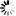# Publication: Remarks on the Critical Behavior of Second Order Additive Invariants in Elementary Cellular Automata

All || By Area || By Year

Title Remarks on the Critical Behavior of Second Order Additive Invariants in Elementary Cellular Automata
Authors/Editors* H. FukÅ
Where published* Fundamenta Informaticae
How published* Journal
Year* 2007
Volume 78
Number 1
Pages 329-341
Publisher
Keywords
We present results of some numerical investigations of second order additive invariants in elementary cellular automata rules. Fundamental diagrams of rules which possess additive invariants are either linear or exhibit singularities similar to singularities of rules with first-order invariant. Only rules whic h have exactly one invariants exhibit singularities. At the singularity, the current decays to its equilibrium value as a power law $t^{\alpha}$, and the value of the exponent $\alpha$ obtained from numerical simulations is very close to $-1/2$. This is in agreements with values previously reported for number-conserving rule s, and leads to a conjecture that regardless of the order of the invariant, exponent $\alpha$ has a universal value of $1/2$.